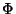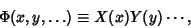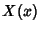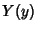## Separation of Variables

A method of solving partial differential equations in a functionand variables,, ... by making a substitution of the formbreaking the resulting equation into a set of independent ordinary differential equations, solving these for,, ..., and then plugging them back into the original equation.

This technique works because if the product of functions of independent variables is a constant, each function must separately be a constant. Success requires choice of an appropriate coordinate system and may not be attainable at all depending on the equation. Separation of variables was first used by L'Hospitalin 1750. It is especially useful in solving equations arising in mathematical physics, such as Laplace's Equation, the Helmholtz Differential Equation, and the Schrödinger equation.Arfken, G. Separation of Variables'' and Separation of Variables--Ordinary Differential Equations.'' §2.6 and §8.3 in Mathematical Methods for Physicists, 3rd ed. Orlando, FL: Academic Press, pp. 111-117 and 448-451, 1985.
Morse, P. M. and Feshbach, H. Separable Coordinates'' and Table of Separable Coordinates in Three Dimensions.'' §5.1 in Methods of Theoretical Physics, Part I. New York: McGraw-Hill, pp. 464-523 and 655-666, 1953.